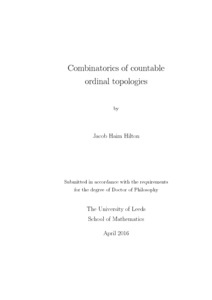# Combinatorics of countable ordinal topologies

Hilton, Jacob Haim (2016) Combinatorics of countable ordinal topologies. PhD thesis, University of Leeds.Preview
Text
Hilton_JH_Mathematics_PhD_2016.pdf - Final eThesis - complete (pdf)
Available under License Creative Commons Attribution-Noncommercial-Share Alike 2.0 UK: England & Wales.

Download (1076Kb) | Preview

## Abstract

We study combinatorial properties of ordinals under the order topology, focusing on the subspaces, partition properties and autohomeomorphism groups of countable ordinals. Our main results concern topological partition relations. Let n be a positive integer, let κ be a cardinal, and write [X] n for the set of subsets of X of size n. Given an ordinal β and ordinals αi for all i ∈ κ, write β →top (αi) n i∈κ to mean that for every function c : [β] n → κ (a colouring) there is some subspace X ⊆ β and some i ∈ κ such that X is homeomorphic to αi and [X] n ⊆ c −1 ({i}). We examine the cases n = 1 and n = 2, defining the topological pigeonhole number P top (αi) i∈κ to be the least ordinal β (when one exists) such that β →top (αi) 1 i∈κ , and the topological Ramsey number Rtop (αi) i∈κ to be the least ordinal β (when one exists) such that β →top (αi) 2 i∈κ . We resolve the case n = 1 by determining the topological pigeonhole number of an arbitrary sequence of ordinals, including an independence result for one class of cases. In the case n = 2, we prove a topological version of the Erd˝os–Milner theorem, namely that Rtop (α, k) is countable whenever α is countable and k is finite. More precisely, we prove that Rtop(ω ω β , k + 1) ≤ ω ω β·k for all countable ordinals β and all positive integers k. We also provide more careful upper bounds for certain small ordinals, including Rtop(ω + 1, k + 1) = ω k + 1, Rtop(α, k) < ωω whenever α < ω2 , Rtop(ω 2 , k) ≤ ω ω and Rtop(ω 2 + 1, k + 2) ≤ ω ω·k + 1 for all positive integers k. Outside the partition calculus, we prove a topological analogue of Hausdorff’s theorem on scattered total orderings. This allows us to characterise countable subspaces of ordinals as the order topologies of countable scattered total orderings. As an application, we compute the number of subspaces of an ordinal up to homeomorphism. Finally, we study the group of autohomeomorphisms of ω n ·m+1 for finite n and m. We classify the normal subgroups contained in the pointwise stabiliser of the limit points. These subgroups fall naturally into D (n) disjoint sets, each either countable or of size 22 ℵ0 , where D (n) is the number of ⊆-antichains of P ({1, 2, . . . , n}). Our techniques span a variety of disciplines, including set theory, general topology and permutation group theory

Item Type: Thesis (PhD) Ordinal topology, countable ordinal, partition relation, pigeonhole principle, Ramsey number, autohomeomorphism group The University of Leeds > Faculty of Maths and Physical Sciences (Leeds) > School of Mathematics (Leeds) > Pure Mathematics (Leeds) uk.bl.ethos.690144 Mr Jacob Haim Hilton 02 Aug 2016 14:38 06 Oct 2016 14:43 http://etheses.whiterose.ac.uk/id/eprint/13608

You do not need to contact us to get a copy of this thesis. Please use the 'Download' link(s) above to get a copy.
You can contact us about this thesis. If you need to make a general enquiry, please see the Contact us page.